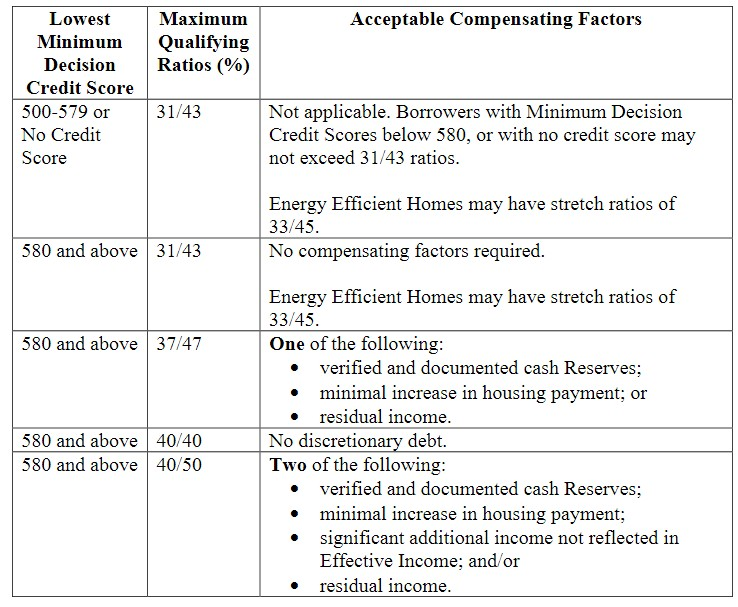﻿ How to calculate debt to income ratio for FHA loan

# How to calculate debt to income ratio for FHA loanLenders use a simple formula to calculate the maximum loan amount. The calculation is known as debt-to-income. In other words, how much monthly debt can the borrower carry in comparison to his or her monthly income?

## Front end ratio

The FHA employs two computations. The first calculation is known as the front end ratio. This computation calculates the maximum mortgage payment. The second calculation, known as the back end ratio, combines the monthly debt and the projected mortgage payment. Let us now look at the payment ratio.

Assume a monthly gross income of \$2,000 and a projected mortgage payment of \$700 (the mortgage payment comprises principle and interest, 1/12 of the yearly real estate taxes, plus homeowners insurance, mortgage insurance, and so on).
Divide \$800 by your monthly salary of \$2,000.
The outcome is 35%.

## Back end ratio

The back end ratio comes next. We'll suppose the borrower's monthly expenses total \$300. Add the monthly expenditures and the anticipated monthly mortgage payment (\$300 + \$700 = \$1,000). Next, divide \$1,000 by the monthly income; the final figure is 50%. (too high).

The acceptable debt-to-income ratio is determined by the applicant's credit score.
Here's a graphic from the FHA underwriting handbook that summarizes the maximum debt-to-income ratios.SOURCE: FHA Handbook 4000.1